Concept Detail
Maths / Linear Equations in Two Variables / Representing an Equation in one variable on a graph
(A Brief Glimpse of ABHYAS Content - Have aLook !!!!)

## Representing an Equation in One Variable on a Graph

An equation in one variable can be easily represented on a number line by plotting the value of the variable on it. We can also represent the equation on a graph by considering the coefficient of the missing variable as zero, and then assuming the different values of the missing variable we can find the solutions and then plot the solutions on the graph.

Illustration: Plot the equation x= 4 on the graph

Solution: Let the coefficient of the missing variable be zero. The equation can be written as  ;   x + 0y = 4

For y=2 , the equation becomes x + 0(2)=4 or x = 4

For y=4 , the equation becomes x + 0(4)=4 or x = 4

For y=6 , the equation becomes x + 0(6)=4 or x = 4

So (4,2), (4,4) and (4,6) are the solution of the equation plotting them on the graph we getWe get a line parallel to Y- Axis

Similarly if we plot the equation y=4,  we get a line parallel to X- Axis

We can further generalize that an equation in one variable represents a line parallel to the axis whose variable is missing in the equation.A Brief Look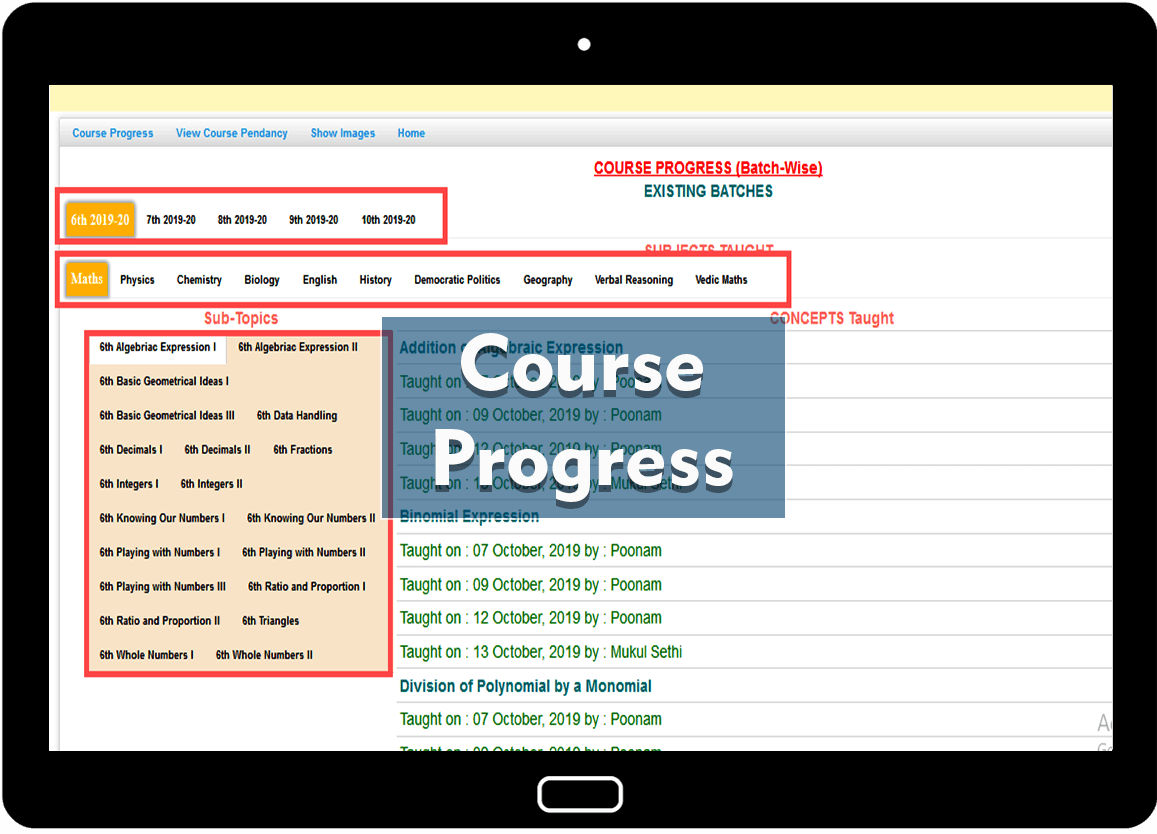Course Progress PANEL
Student can view their progress from this panel.
Self Study
Student can learn from Self Study panel. Concept notes and Video will appear their to learn concept. Exam will be held on the basis of concepts learn.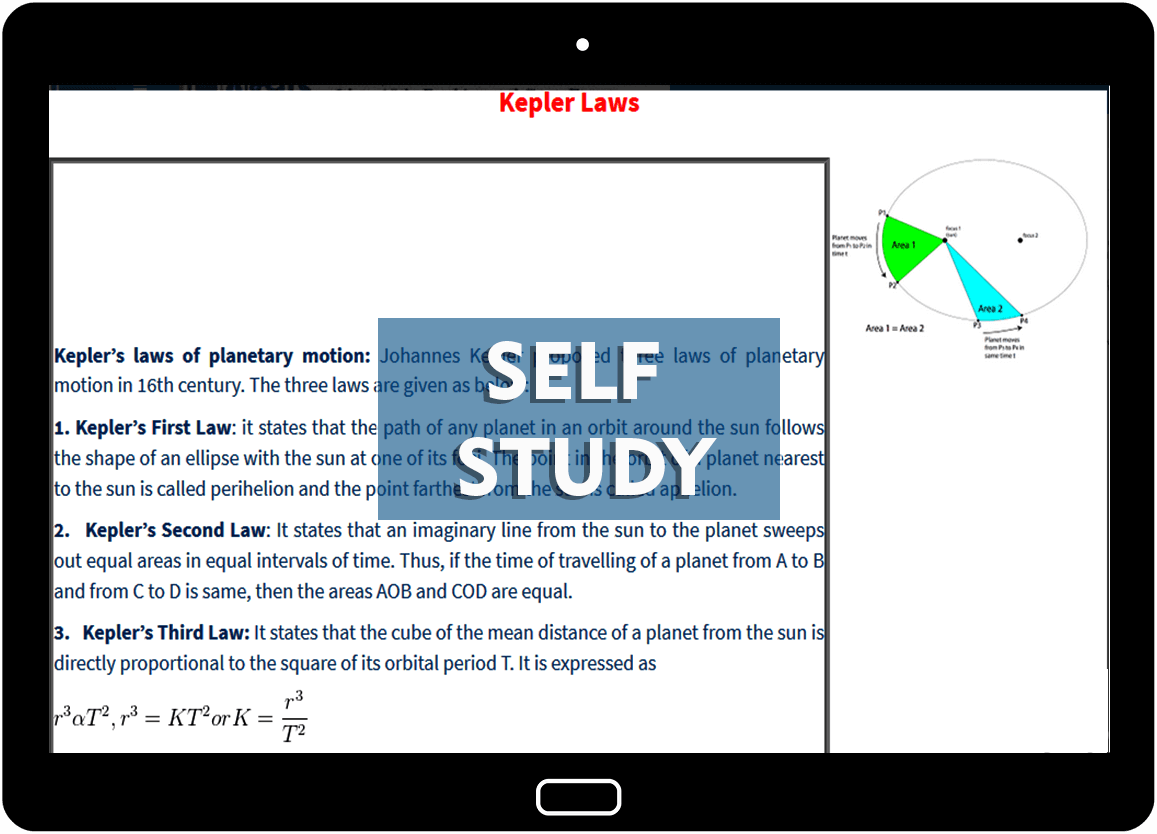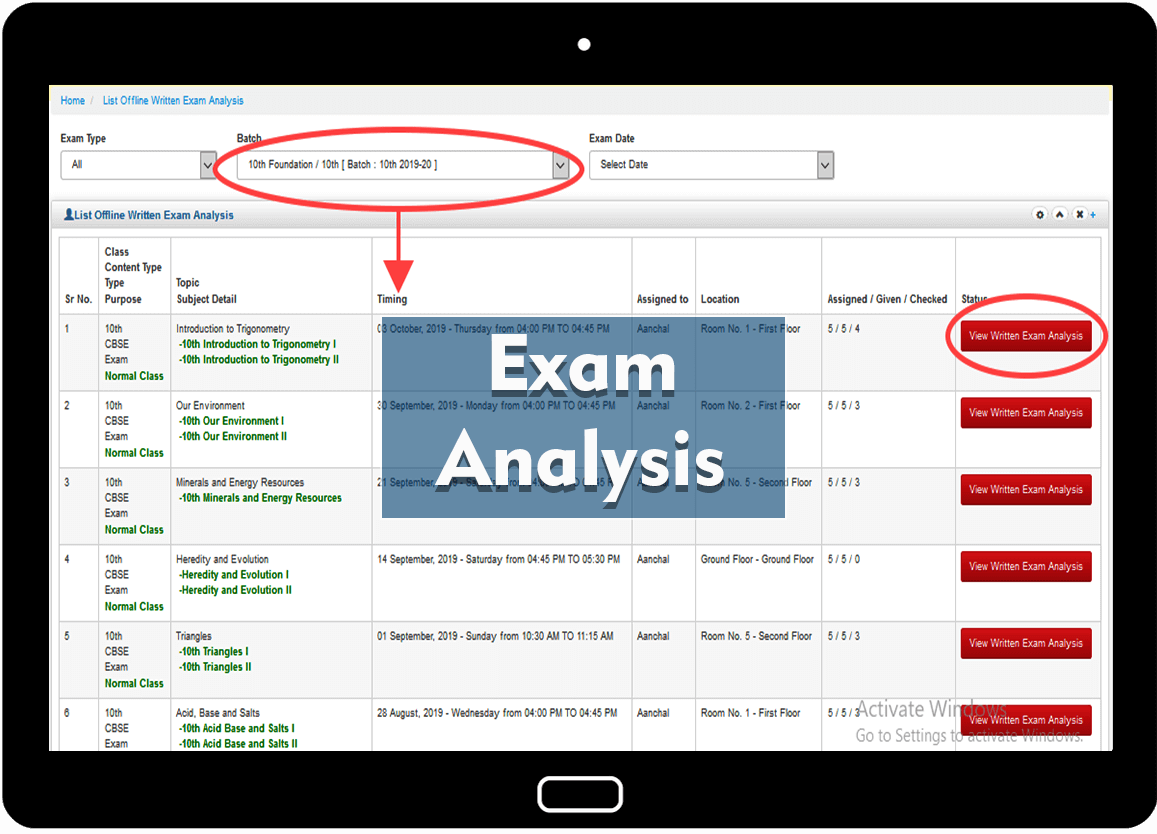Exam Evaluation PANEL
Detailed Exam Analysis for Offline and online exams will be Available in Student dashboard. Offline exams will be held for in-campus students only.
Class Notes PANEL
Student can view / download Class Notes assigned to them. Request can also be sent for elapsed Notes.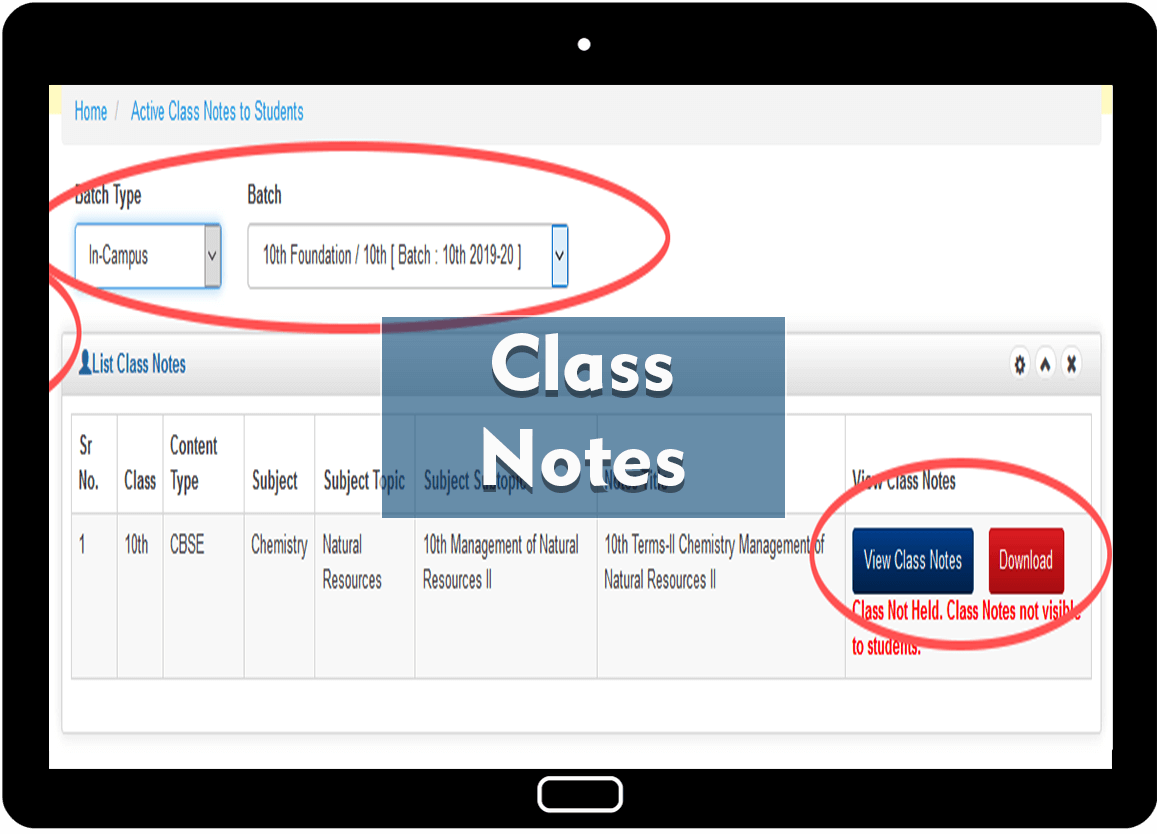REPORT CARD
Report Card of each student is visble in Student Panel for WISE-FA, WISE-PT, WISE-Testing, Written Exams, Attendance, etc.
STUDENT PANEL
Ranking amongst the students of the class are visible at any time. Students rank will automatically based on the exams given by the students and score.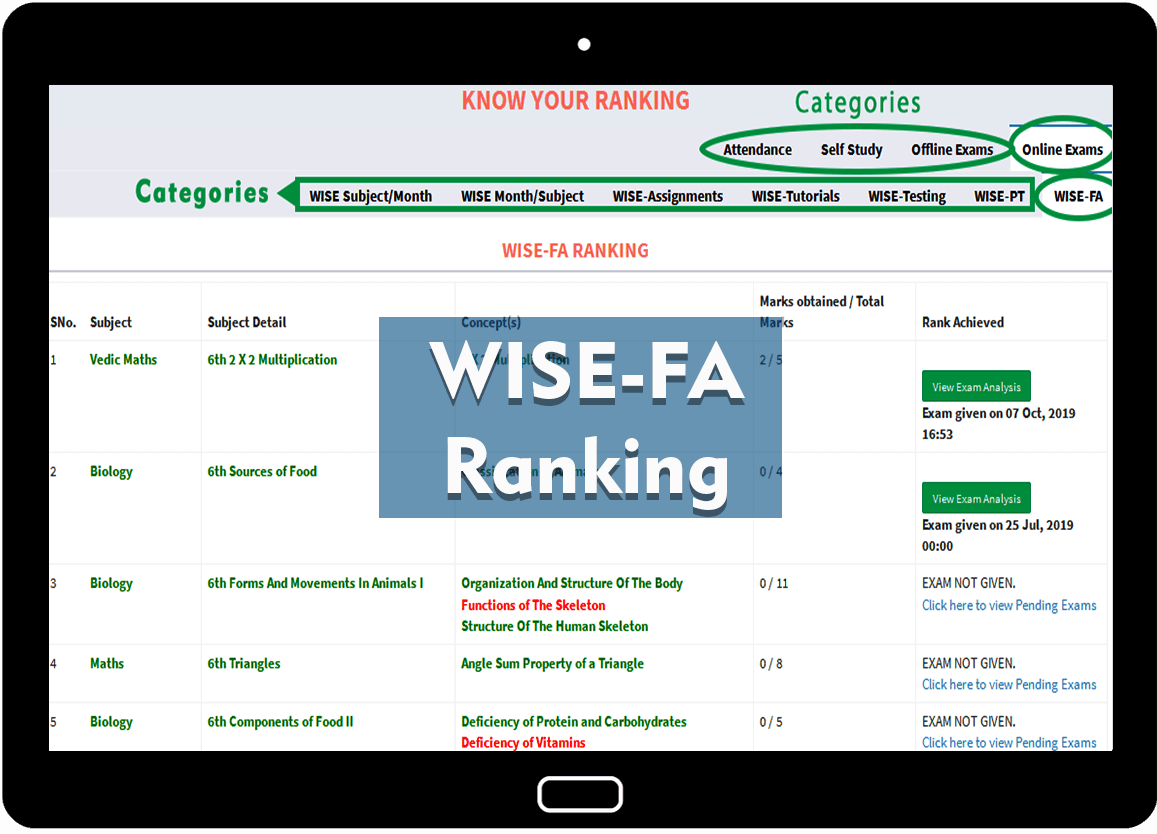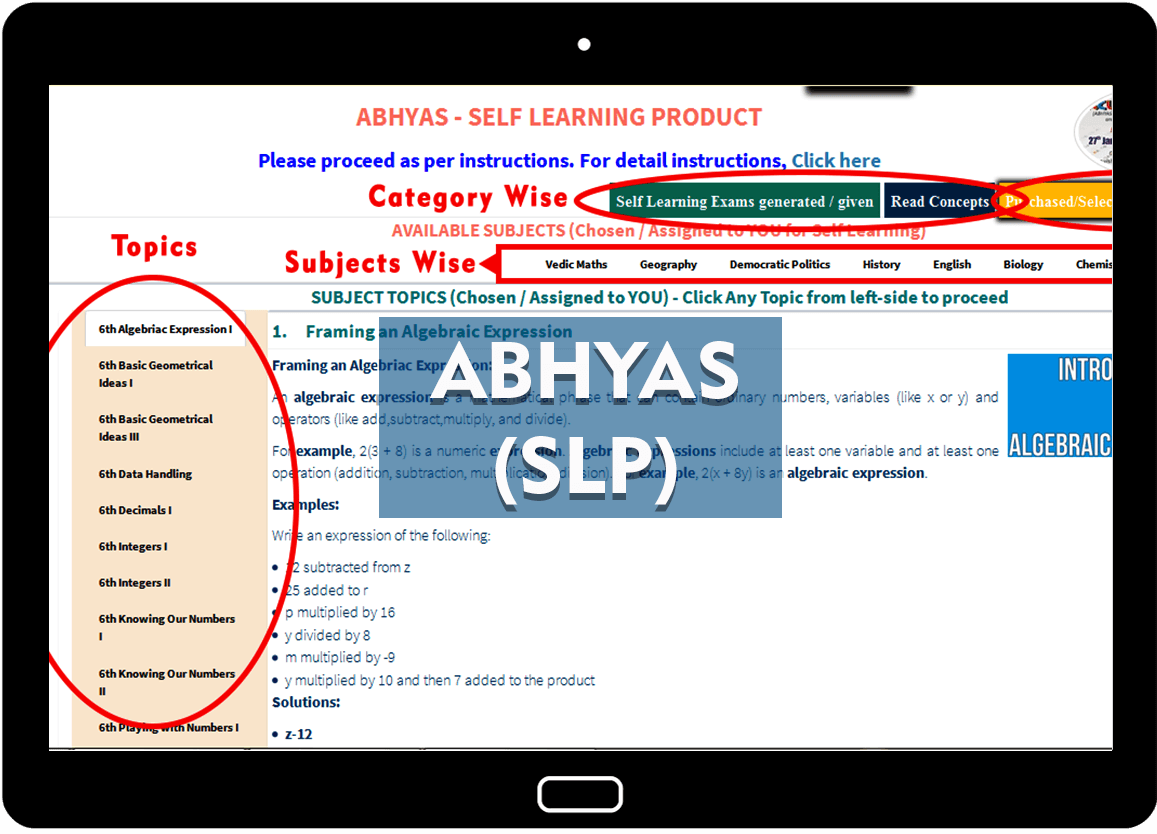SELF LEARNING PANEL
Self Learning panel to know topics/concepts read and exams given. Detail analysis provided to students for exams given. Remedial papers will be generated on student choice for Weak Concepts.
TEST GENERATOR
Test generator to generate test for concepts taught and pending.STUDENT DASHBOARD
To know Online and Offline exams given / pending with graphical view.